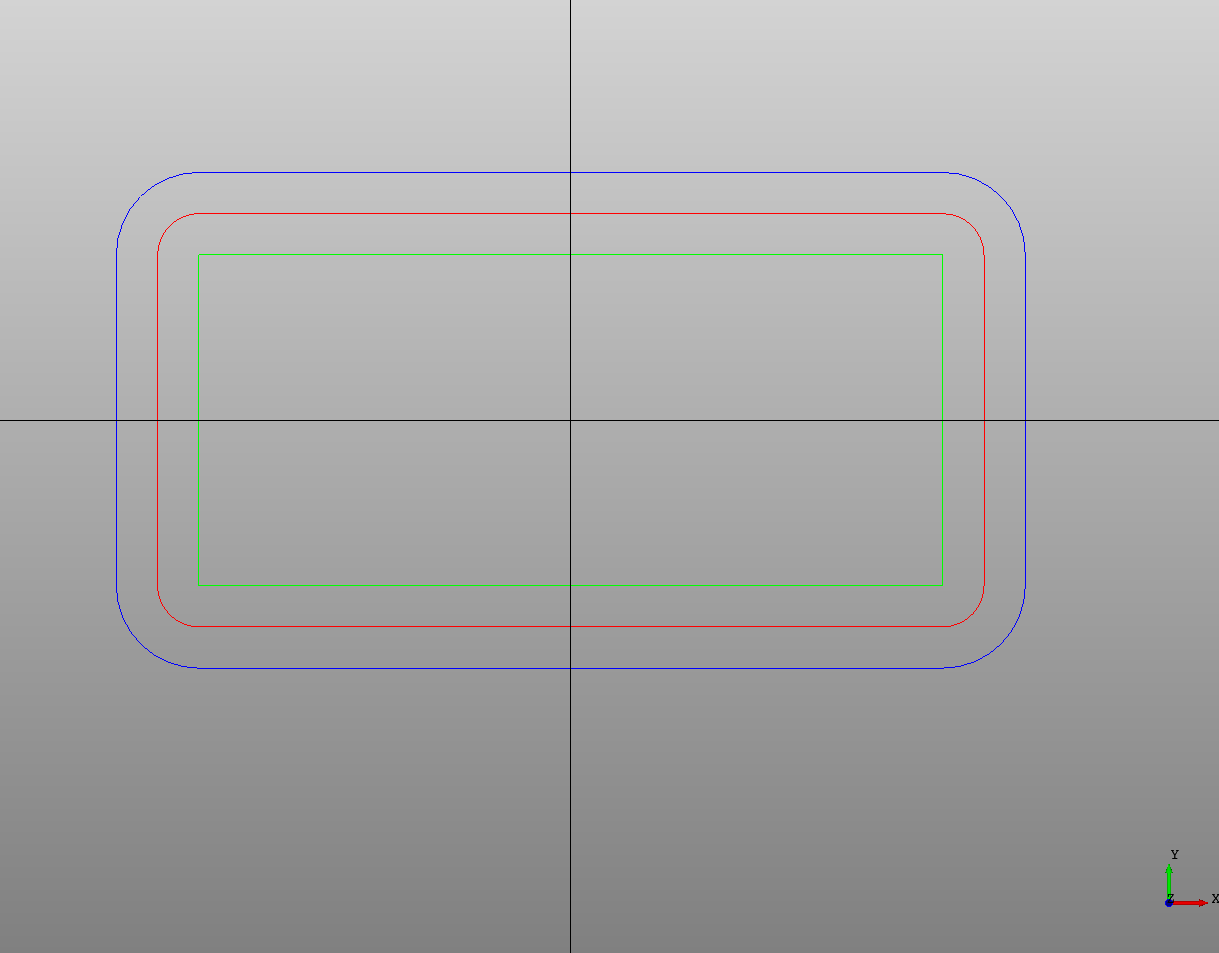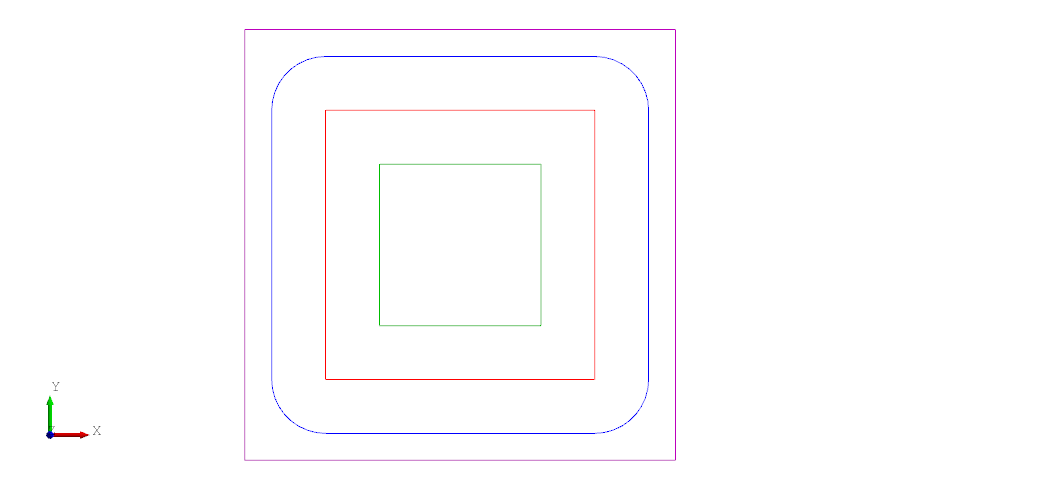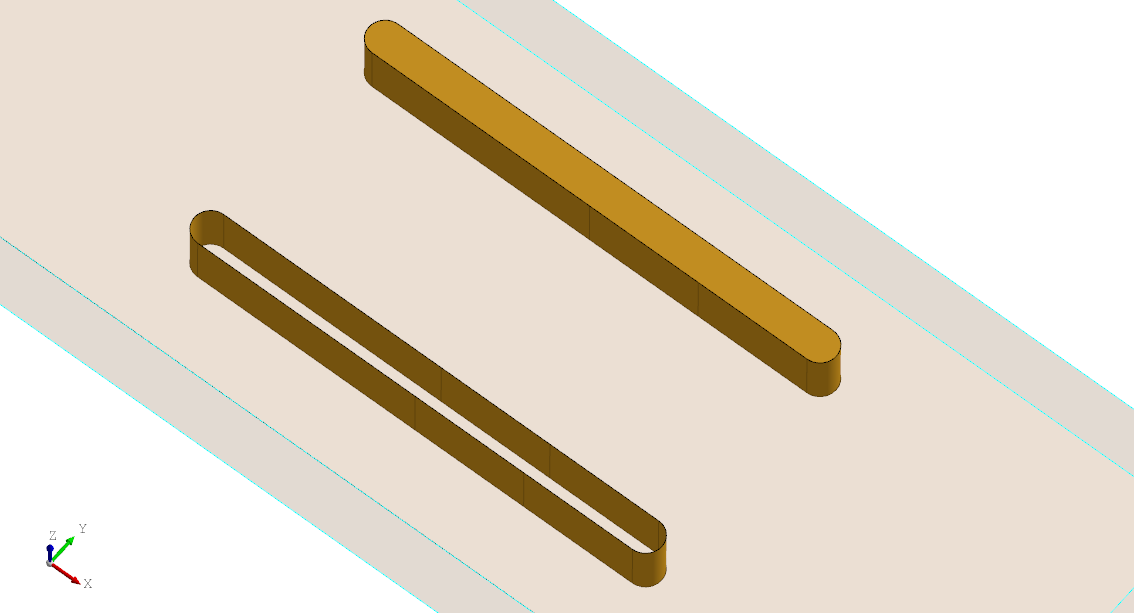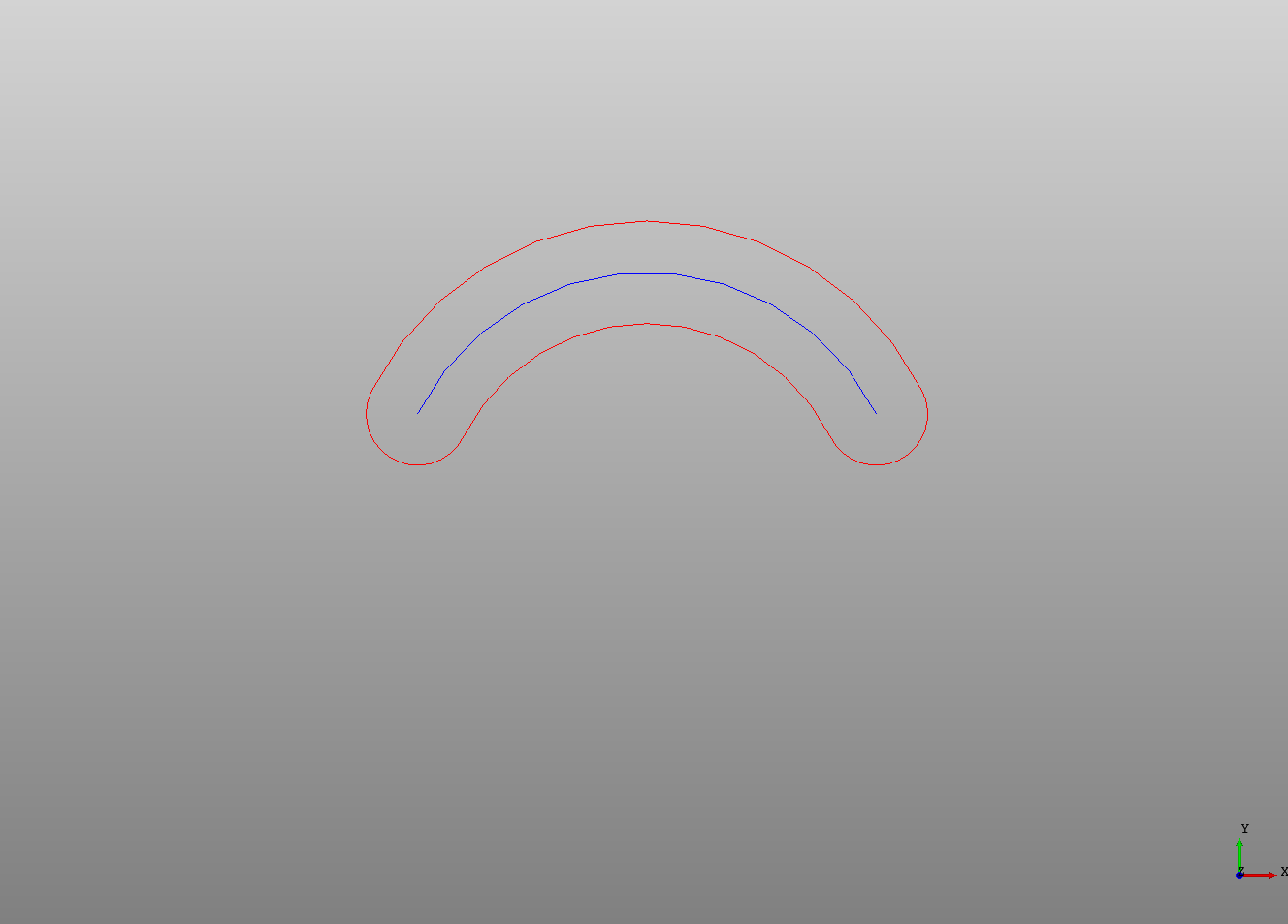## Offset Curves

The `Offset` declaration can be used to create a planar offset curve or face from an edge that is offset by a given positive or negative `offset` distance.

``````# Created in DeclaraCAD

enamldef Assembly(Part):
Rectangle:  r1:
color = 'red'
# Center on origin
position = (-width/2, -height/2)
height = 10
width = 20
rx = 1
Offset: o1:
color = 'blue'
shape = r1
offset = 1
Offset: o2:
color = 'lime'
shape = r1
offset = -1

# For reference
Line: xaxis:
color = 'black'
direction = (1, 0)
Line: yaxis:
color = 'black'
direction = (0, 1)
``````Notice how the positive and negative offset distances produce different shapes. This also depends on the "path direction" of the wire.

Attempting to use `Offset` on shapes that are not in the same plane will give an error. Also offsetting a face with holes in it can also give errors.

Note: The shape being offset must be able to be converted to a plane (it must have a normal). A single line segment for example has no normal. To offset a single line segment split it into two segments and make a wire from it.

##### Normal distance

An offset and shift along the plane normal can be completed in one `Offset` operation by specifying the `normal_distance`. For example a circle with a normal of the Z axis with `normal_distance = 1` will be shifted "up" by 1 in the Z direction.

##### Join type

In certain situations you might not want the offset curve to produce arcs when expanding corners. The `join_type` defaults to `arc` but can be set to either `tangent` or `intersection` to change how the offset curves are joined together. In the screenshot below the blue square has a positive offset from the red square with the default `join_type = "arc"` while the purple square has a positive offset with `join_type = "intersection"`. The green square has a negative offset and is unchanged.##### As face

Use `as_face = True` to convert the result into a face otherwise it will be a wire. This allows you to skip an extra `Face` node. The following example shows the difference when extruding a face vs a wire.

``````enamldef SimplePart(Part):

Box: stock:
transparency = 0.8
position = (-dx/2, -dy/2,  -dz)
dx = 100
dy = 40
dz = 10
Extrude:
vector = (0, 0, -stock.dz/2)
Offset:
offset = 1.5
as_face = True
Polyline:
points = [(-20, 10), (0, 10), (20, 10)]
Extrude:
vector = (0, 0, -stock.dz/2)
Offset:
offset = 1.5
Polyline:
points = [(-20, -10), (0, -10), (20, -10)]
``````## Slots

An `Offset` can be used on to create slots to cut out from a part. Just draw the path the slot should follow and set the offset to the radius of the slot. If a shape is added as a child of the Offset that shape will be offset.

``````# Created in DeclaraCAD

enamldef Assembly(Part):
Offset:
color = 'red'
offset = 1
Arc: arc: